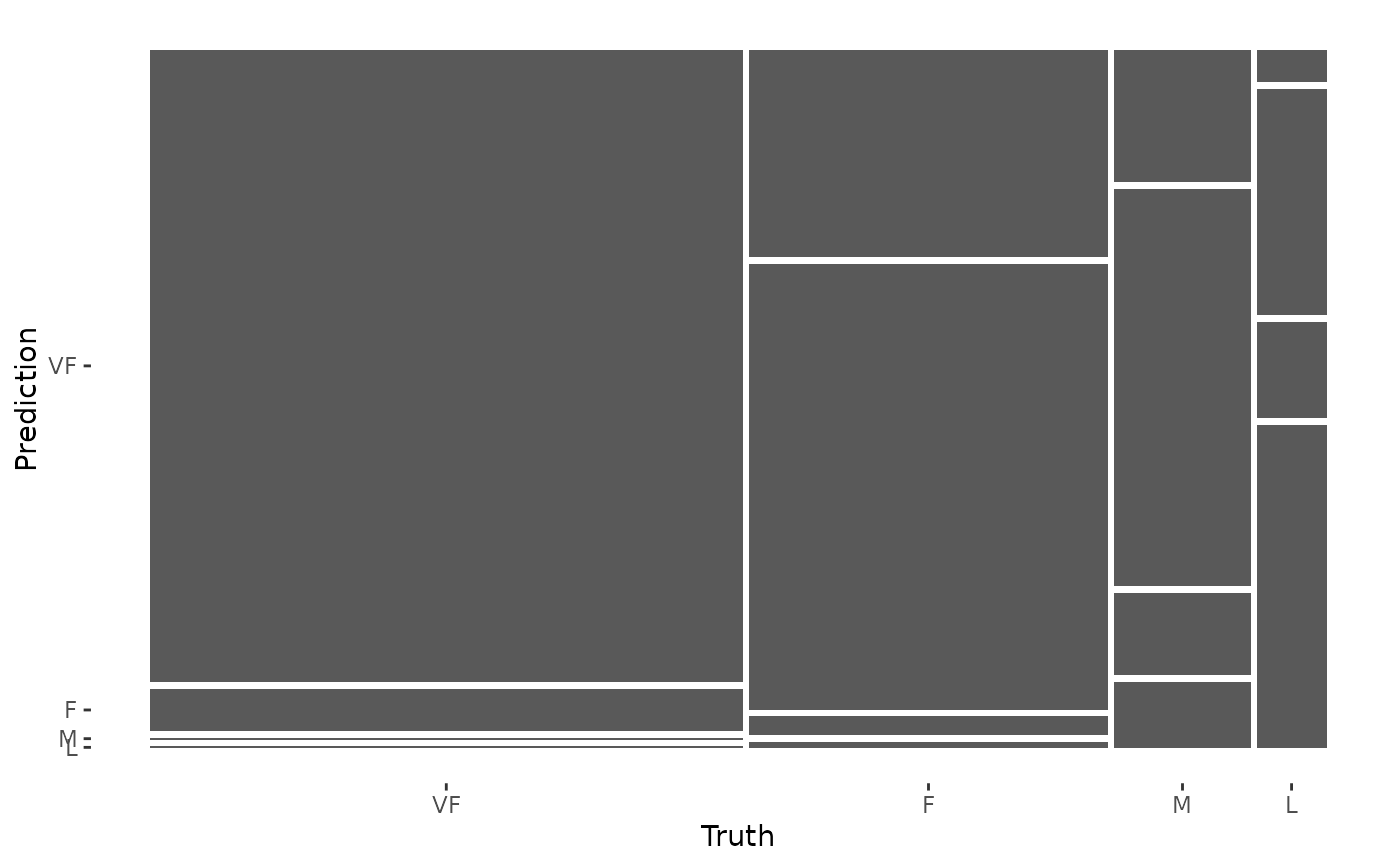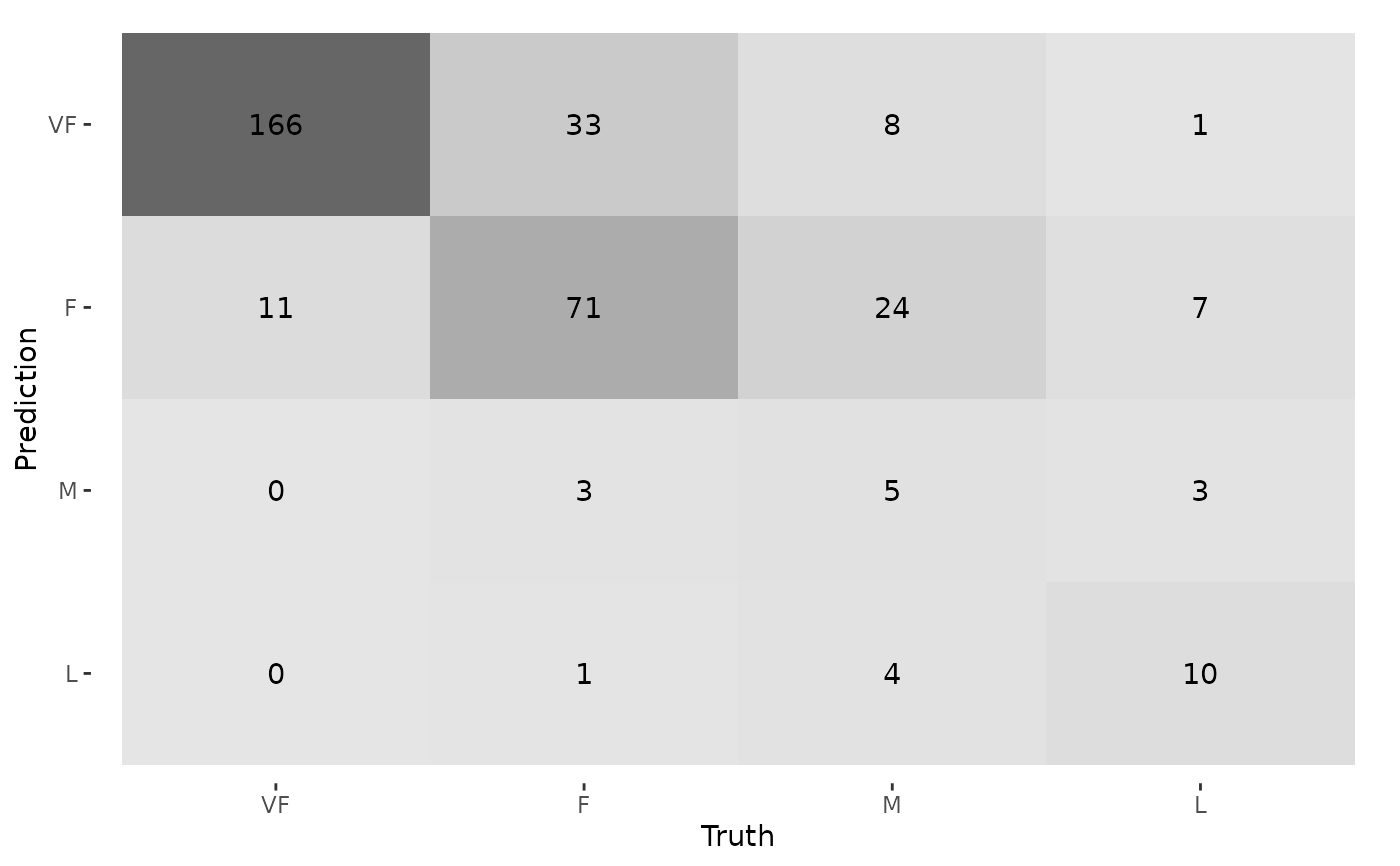Calculates a cross-tabulation of observed and predicted classes.

## Usage

conf_mat(data, ...)

# S3 method for data.frame
conf_mat(
data,
truth,
estimate,
dnn = c("Prediction", "Truth"),
case_weights = NULL,
...
)

# S3 method for conf_mat
tidy(x, ...)

## Arguments

data

A data frame or a base::table().

...

Not used.

truth

The column identifier for the true class results (that is a factor). This should be an unquoted column name although this argument is passed by expression and supports quasiquotation (you can unquote column names). For _vec() functions, a factor vector.

estimate

The column identifier for the predicted class results (that is also factor). As with truth this can be specified different ways but the primary method is to use an unquoted variable name. For _vec() functions, a factor vector.

dnn

A character vector of dimnames for the table.

case_weights

The optional column identifier for case weights. This should be an unquoted column name that evaluates to a numeric column in data. For _vec() functions, a numeric vector.

x

A conf_mat object.

## Value

conf_mat() produces an object with class conf_mat. This contains the table and other objects. tidy.conf_mat() generates a tibble with columns name (the cell identifier) and value (the cell count).

When used on a grouped data frame, conf_mat() returns a tibble containing columns for the groups along with conf_mat, a list-column where each element is a conf_mat object.

## Details

For conf_mat() objects, a broom tidy() method has been created that collapses the cell counts by cell into a data frame for easy manipulation.

There is also a summary() method that computes various classification metrics at once. See summary.conf_mat()

There is a ggplot2::autoplot() method for quickly visualizing the matrix. Both a heatmap and mosaic type is implemented.

The function requires that the factors have exactly the same levels.

summary.conf_mat() for computing a large number of metrics from one confusion matrix.

## Examples

library(dplyr)
data("hpc_cv")

# The confusion matrix from a single assessment set (i.e. fold)
cm <- hpc_cv %>%
filter(Resample == "Fold01") %>%
conf_mat(obs, pred)
cm
#>           Truth
#> Prediction  VF   F   M   L
#>         VF 166  33   8   1
#>         F   11  71  24   7
#>         M    0   3   5   3
#>         L    0   1   4  10

# Now compute the average confusion matrix across all folds in
# terms of the proportion of the data contained in each cell.
# First get the raw cell counts per fold using the tidy method
library(tidyr)

cells_per_resample <- hpc_cv %>%
group_by(Resample) %>%
conf_mat(obs, pred) %>%
mutate(tidied = lapply(conf_mat, tidy)) %>%
unnest(tidied)

# Get the totals per resample
counts_per_resample <- hpc_cv %>%
group_by(Resample) %>%
summarize(total = n()) %>%
left_join(cells_per_resample, by = "Resample") %>%
# Compute the proportions
mutate(prop = value/total) %>%
group_by(name) %>%
# Average
summarize(prop = mean(prop))

counts_per_resample
#> # A tibble: 16 × 2
#>    name         prop
#>    <chr>       <dbl>
#>  1 cell_1_1 0.467
#>  2 cell_1_2 0.107
#>  3 cell_1_3 0.0185
#>  4 cell_1_4 0.00259
#>  5 cell_2_1 0.0407
#>  6 cell_2_2 0.187
#>  7 cell_2_3 0.0632
#>  8 cell_2_4 0.0173
#>  9 cell_3_1 0.00173
#> 10 cell_3_2 0.00692
#> 11 cell_3_3 0.0228
#> 12 cell_3_4 0.00807
#> 13 cell_4_1 0.000575
#> 14 cell_4_2 0.0104
#> 15 cell_4_3 0.0144
#> 16 cell_4_4 0.0320

# Now reshape these into a matrix
mean_cmat <- matrix(counts_per_resample$prop, byrow = TRUE, ncol = 4) rownames(mean_cmat) <- levels(hpc_cv$obs)
colnames(mean_cmat) <- levels(hpc_cv\$obs)

round(mean_cmat, 3)
#>       VF     F     M     L
#> VF 0.467 0.107 0.018 0.003
#> F  0.041 0.187 0.063 0.017
#> M  0.002 0.007 0.023 0.008
#> L  0.001 0.010 0.014 0.032

# The confusion matrix can quickly be visualized using autoplot()
library(ggplot2)

autoplot(cm, type = "mosaic")autoplot(cm, type = "heatmap")